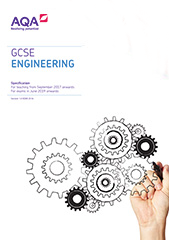# Appendix 1: Mathematical understanding

A grasp of a range of mathematical concepts and skills is key to success in engineering. This section outlines the equations and mathematical skills students will be expected to recall and use within the exam. Not all of these equations and skills will be tested in every exam series but students may be asked to use any combination of equations and skills.

The mathematical content has been developed in order to support teaching of GCSE Engineering and should not be seen as an additional element. The skills in mathematics are not intended to be taught separately to the main content, but integrated into schemes of work. The skill groups are indicated in the subject content, using the references E1 (Equation 1), E2 (Equation 2) or M1 (Mathematical Skill 1), M2 (Mathematical Skill 2) etc.

Students should recognise, carry out calculations and be able to communicate using the following SI units: millimetres (mm), metres (m), kilograms (Kg), tonnes (T), newtons (N), volt (V), ohm (Ω); and the following SI multipliers: p, n, µ, m, k, M, G and T.

Students should also have knowledge and understanding of the following conversion of units:

• mm to cm

• cm to m

• litres to ml, to cm3, and to mm3

• kg to tonnes

• weight to mass.

## Equations

Through their work in engineering, students will be expected to apply relevant knowledge, skills and understanding from Key Stage 3 and 4 courses in mathematics.

Equation number Description Equation
E1 Area of a rectangle A=L×W
E2 Volume of a cuboid V = L × W × H
E3 Area of a circle Ac=πr2
E4 Volume of a cylinder Vc=Ac×L
E5 Area of a triangle At=½(B×H)
E6 Density = mass/volume ρ=m/v
E7 Stress = force/cross-sectional area σ =F/A
E8 Strain = change in length/original length ε=δl/l
E9 Young’s modulus = stress/strain E=σ/ε
E10 Pressure = force/area P=F/A
E11 Factor of safety = material strength/ yield stress FoS=σy/L
E12 Ohm’s law: current = voltage/resistance I=V/R
E13 Series resistance Rt = R1 + R2
E14 Ratio of simple gears: Gear ratio = Number of teeth on driven gear/number of teeth on driver gear

## Mathematical skills

### M1 – Arithmetic and numerical computation

Mathematical skill number Description of skill
M1.1 Recognise and use expressions in decimal form
M1.2 Recognise and use expressions in standard index form
M1.3 Perform calculations using time and cost
M1.4 Use ratios, fractions and percentages
M1.5 Calculate squares and square roots
M1.6 Calculate angles of a triangle using trigonometry
M1.7 Use Pythagoras’ theorem

### M2 – Handling data

Mathematical skill number

Description of skill

M2.1 use an appropriate number of significant figures
M2.2 find arithmetic means
M2.3 make order of magnitude calculations
M2.4 collection, organisation and presentation of data

### M3 – Algebra

Mathematical skill number Description of skill
M3.1 understand and use the symbols =, <, ≤, ≥, >, ±, α, ~
M3.2 change the subject of an equation
M3.3 substitute numerical values into algebraic equations using appropriate units for physical quantities
M3.4 solve simple algebraic equations

### M4 – Graphs

Mathematical skill number Description of skill
M4.1 translate information between graphical and numeric form
M4.2 plot two variables from experimental or other data
M4.3 draw an appropriate trend line onto plotted data
M4.4 determine the slope of a graph
M4.5 interpret data presented in graphical form